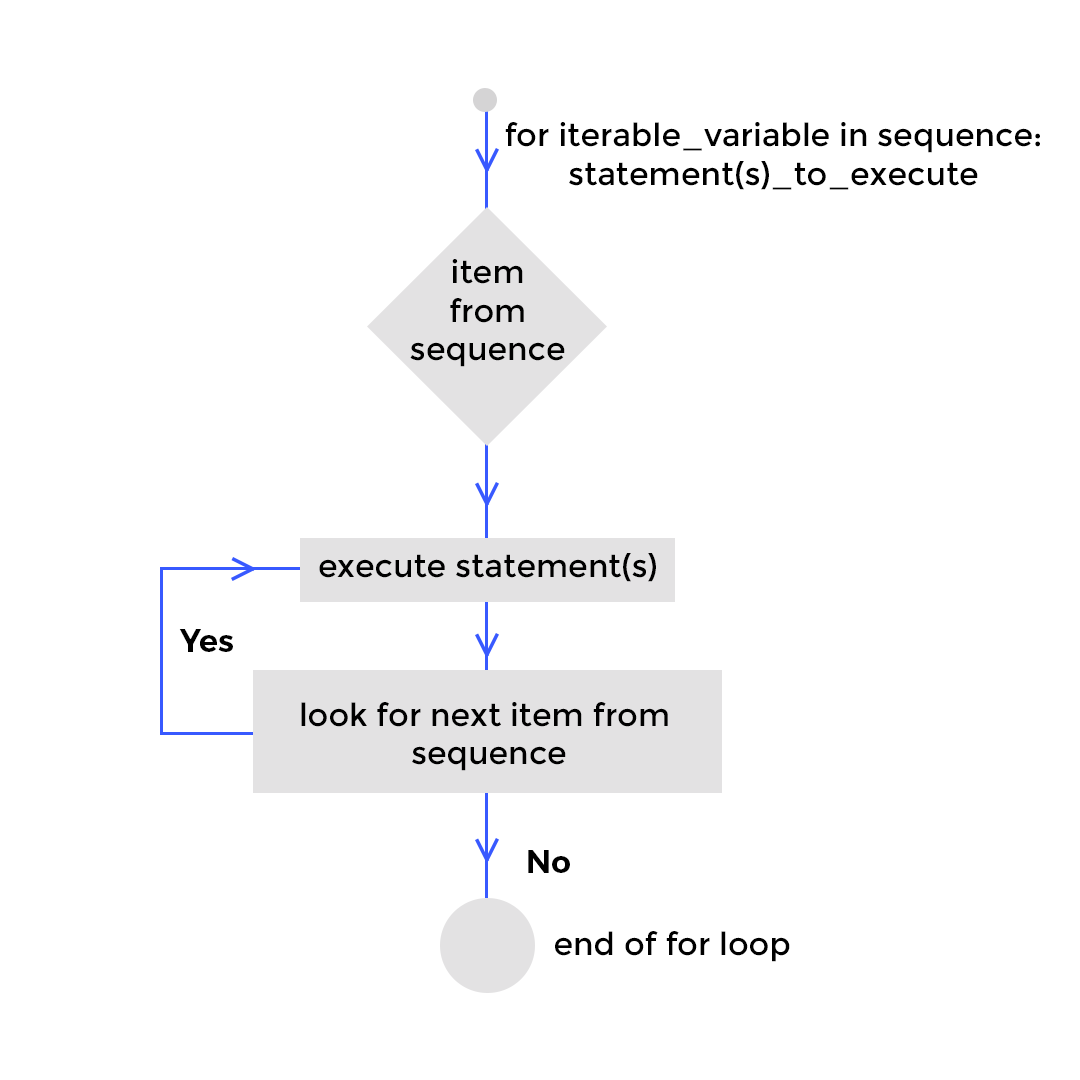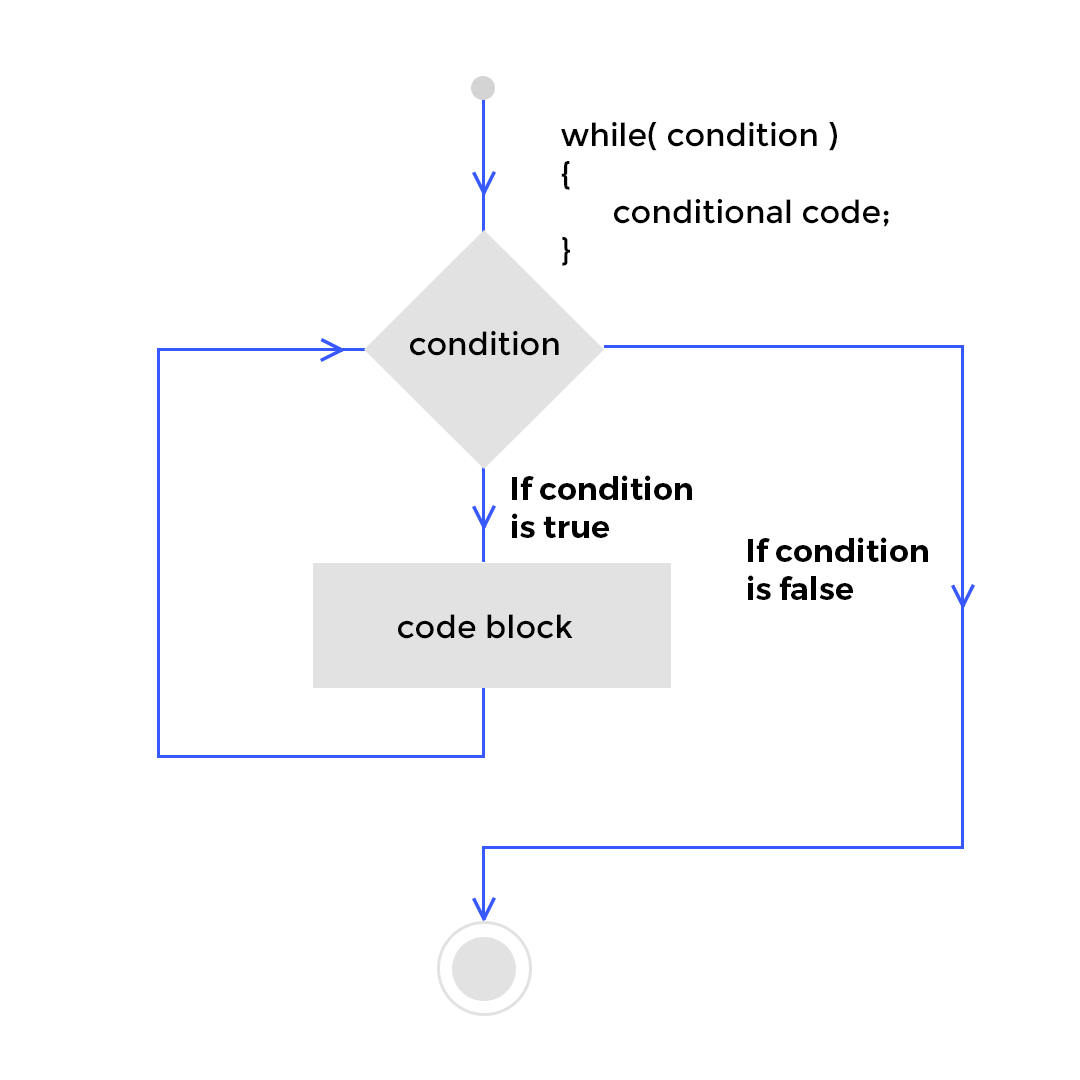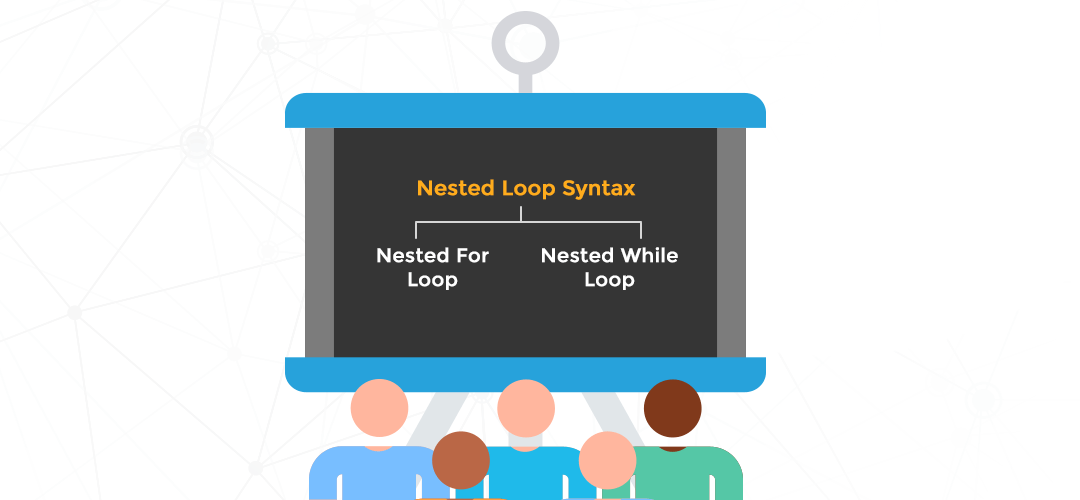# How Well Do You Know Loops in Python – Python Loop (GUIDE)In continuation of the previous post about Python Data Types, we proceed with the loop statements in Python. In this article, we are going to talk about the Python Loop – the For and While loops, their syntaxes with some examples, how we can use Else statements in these loops and how to create nested loops. We will also talk about loop control statements to exercise greater control over the loops.

## For Loop –A for loop acts as an iterator in Python Loop. Objects that a for loop can iterate over include strings, lists, tuples, and built-in iterables for dictionaries, such as its keys or values. The loop goes through all items that are in a sequence or any other iterable item and executes the statements mentioned within the loop.

Here’s the general format for a for loop in Python:

## For Loop Syntax –

```for iterable_variable in sequence:
statements_to_execute```

### For Loop Block Diagram –

When a for loop is encountered, the sequence is executed first if it is an expression. Then, the first item in the sequence is being assigned to the iterable_variable and the statements to execute is being performed. The loop progresses by picking up all the value in sequence one by one, executing the statement block until there is no more value to be assigned from the sequence to the iterable_variable.### For Loop examples:

#### Example 1 – For Loop on a list of strings –

```school_names = ['DPS', 'DAV', 'SDJBV', 'SJV']
i=0
for item in school_names:
item = item + " is a good school"
school_names[i] = item
i = i + 1
print(school_names)```

In the above code, we are working on a list of string elements which is the sequence. The item is our iterable_variable and the statement block concatenates ” is a good school” to each item value and saves it as the elements of the list school_names.

#### Example 2 – For Loop on a list of numbers –

```list1 = [1,2,3,4,5,6,7,8,9,10,11]
for i in range(len(list1)):
if i%2==0:
print(list1[i])```
In the above code, we are printing all the elements from a list of numbers where its index position is even.

### For Loop with Else Clause –

Else statements are usually used with an If statement which we find in all the programming languages. A very interesting feature of Python is that we can use an Else clause in association with the For loop.

Let us first take a look at the traditional if-else conditional statement within a for loop. The code is provided below.

```numbers = [1,2,3,4,'q',3,2,4,2,3,2,1]

for num in numbers:
if type(num) == str:
print ('the list contains a string')
break
else:
print ('the list does not contain any string')```
```The output is :

the list does not contain any string
the list does not contain any string
the list does not contain any string
the list does not contain any string
the list contains a string```

The code checks for the presence of a string value in the sequence list named numbers. For the first four elements which are numeric, the loop executes the else statement and prints the output. When the fifth element string value is found, the if statement becomes true, the consecutive print statement is executed and the break command causes the end of the loop. By observing the

Now let us look at the Else clause after the For loop unique to Python. Here is the code.

```numbers = [1,2,3,4,'q',3,2,4,2,3,2,1]

for num in numbers:
if type(num) == str:
print ('the list contains a string')
break
else:
print ('the list does not contain any string')

The output is:

the list contains a string```

The above code simply checks for a string element in the list. When this condition matches, it executes the print statement and breaks out of the loop. This also renders the Else clause useless. If the if condition is not met, only then the Else clause is executed.

Please Note – The else block just after for is executed only when the loop is NOT terminated by a break statement.

### Single line For Loop –

`[print(i) for i in range(5,100,5)]`

## While Loop –The while loop executes the statements as long as the given condition for the loop is true. When the While condition turns false, the next command after the loop is executed.

### While Loop Syntax –

```while (condition):
conditional code
```

### Block Diagram for a While Loop-### While Loop Example –

```numbers = [1,2,3,4,'q',3,2,4,2,3,2,1]
i =len(numbers)
while(type(numbers[len(numbers)-i]) != str):
print(numbers[len(numbers)-i])
i-=1```

The While loop traces data types of each element in the list and prints the non-string values. The loop terminates at the first finding of a string.

### While Loop with Else –

```numbers = [1,2,3,4,'q',3,2,4,2,3,2,1]
i =len(numbers)
while(type(numbers[len(numbers)-i]) != str):
print(numbers[len(numbers)-i])
i-=1
else:
print("Found a string at index", len(numbers)-i)```
The code has an else statement that will execute only when the While loop condition becomes False. At index 4, the string data type element is found, the While condition turns False consequently executing the Else statement.

## Nested Loops –When we structure a loop within a Python loop, we create a nested loop. Such a loop can comprise of a For loop within another For loop, a While loop within another While loop, or a mix of a For and a While loop.

#### Nested For Loop –

```list1 = [1,2,3,4,5,6,7,8,'a',5]
list2 = [2,4,6,8,'a']
count = 0
for item1 in list1:
for item2 in list2:
if (item1==item2):
count = count + 1
if (count >1):
print("We have" , count , "elements matching")
else:
print("No elements are matching")```

The code matches elements between two lists, counts then umber of matching elements, and prints the result.

#### Nested While Loop –

```i = [1,2,3,4,5,6,7,8]
j = [10,11,12,13,14,15,16,17]
y=0
x=len(j)-1
while x>=0:
while y<len(j)-1:
print(i[x], ",", j[y])
y = y + 1
x = x - 1```

The code above simply prints elements of the first list in ascending order of its index while prints in reverse order for the second list.

Can you think of a case where we construct a Nested loop with a For and a While together? Do let us know in the comment section.

## Loop Control Statements –

Loop control statements give us the power to change the execution from its normal flow or sequence in a way we want. Python supports the following control statements.Continue Statement – It returns the control to the beginning of the loop.

Break Statement – It takes the control out of the loop

Pass Statement – We use pass statement to write empty loops.

Let us see how we can solve the below problem by using the control statements.

Problem Statement

Write a program that repeatedly prompts the user for an integer. If the integer is even, print the integer. If the integer is odd, don’t print anything. Exit the program if the user enters the integer 99.

Solution code –

```while True:

try:
x = int(input("enter a number - "))
if(x%2==0):
print("The integer",x,"is even")
continue
elif(x%2!=0):
pass
elif(x==99):
break

except:
print ("Not an integer")
continue

```

## Conclusion –

In this article, we have given a detailed explanation of all the Python Loops – For, While, and Nested loops with interesting yet simple examples to get a clear understanding. I hope you enjoyed reading and learn by practicing. Stay tuned for more such tutorials. Happy Learning!!